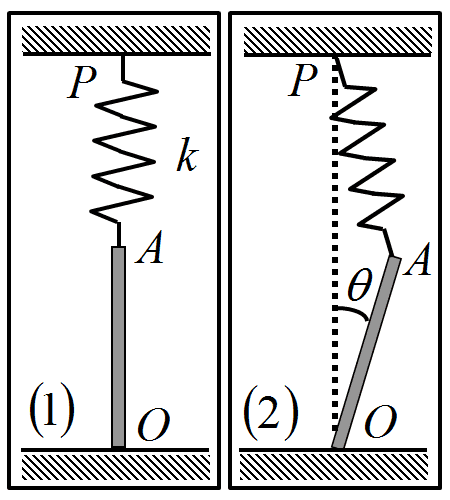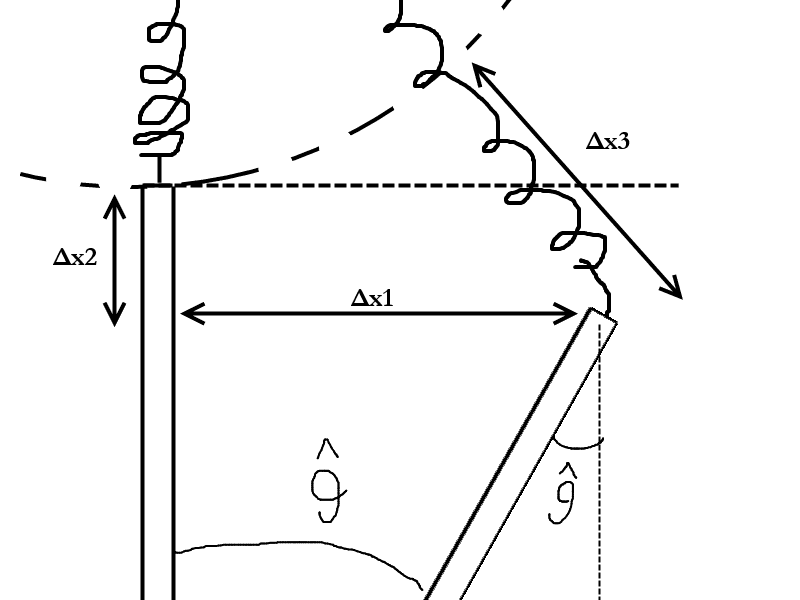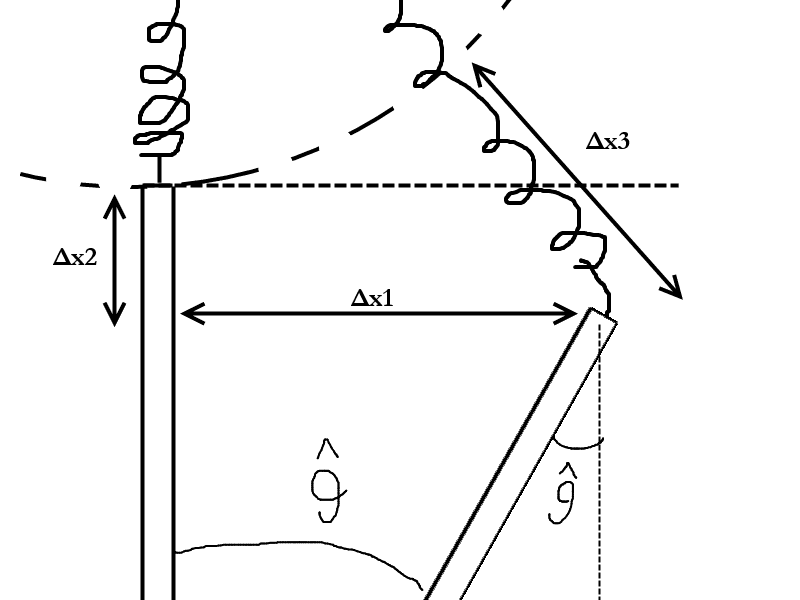# Bar and spring oscillation problem (1 Viewer)

### Users Who Are Viewing This Thread (Users: 0, Guests: 1)

#### slurd

1. The problem statement, all variables and given/known dataA bar with length $l$ and homogeneous mass distribution (mass: $m$) can rotate around its fixed point $O$.The other edge of the bar is connected to the spring in the figure of constant $k$ and length $l$, and the spring is fixed by its one edge at point $P$. The system is in the homogeneous gravitational field of the Earth.

i)find the positions of equilibrium (stable and unstable)
ii)show that for a small $\widehat{\theta}$ the motion that will result is approximately harmonic oscillation.

2. Relevant equations

$kl = mg$

3. The attempt at a solution
First of all, the only equilibrium must be the stable one with θ=0rad since kl=mg.

I tried calculating the Δx (in the length of the spring) using simple triangle geometry but the result is very messy and doesn't get me anywhere.

This is not like any other oscillation problem I have encountered so pardon my ignorance.

#### voko

What about $\theta = 180^\circ$? Is that equilibrium?

Regarding the messy trigonometry, it is OK. Do that, and then simplify on the assumption that the angle is small.

#### slurd

What about $\theta = 180^\circ$? Is that equilibrium?
There will still be a component on the x-axis of the spring's hooke's law force that will point to the left, don't I need $∑F=0$ for equilibrium?
Regarding the messy trigonometry, it is OK. Do that, and then simplify on the assumption that the angle is small.
I presume that it is messy because I am not doing it correctly. I can't simplify the cosθ and sinθ because the Δx ends up 0.

#### voko

There will still be a component on the x-axis of the spring's hooke's law force that will point to the left, don't I need $∑F=0$ for equilibrium?
How so? 180° is vertically down.

I presume that it is messy because I am not doing it correctly. I can't simplify the cosθ and sinθ because the Δx ends up 0.
Unless you show what you do, we can't help much.

#### slurd

How so? 180° is vertically down.
Οοps, was thinking of 90 degrees. 180 is not possible, since the bar would be stopped by the floor's surface.

Unless you show what you do, we can't help much.
Okay I found what was wrong with the Δx=0 equation. But now I'm at this:$Δx_{1} = lsinθ$
$Δx_2 = l-lcosθ$
pythagorean theorem:
$Δx_{1}^{2} + (l+Δx_{2})^{2}=(l+Δx_{3})^{2}$
solve for Δx3:
$Δx_{3} = \sqrt{l^{2}sin^{2}θ+(l+l-lcosθ)^{2}} - l$
$Δx_{3} = l^{4}\sqrt{sin^{2}θ + (2-cosθ)^{2}} - l$
for small $θ$s:
$Δx_{3} = l^{4}\sqrt{0 + (2-1)^{2}} -l$
$Δx_{3} = l^{4}\sqrt{1}-l=l^{4}-l$
The result can't be that big for really small $θ$s.

#### voko

Οοps, was thinking of 90 degrees. 180 is not possible, since the bar would be stopped by the floor's surface.
There is no floor in the formulation and it is not entirely clear from the picture whether the bar can rotate all the way down. I recommend that you consider this case as well. You can add a note saying it was not completely clear whether it is a possible configuration.

Okay I found what was wrong with the Δx=0 equation. But now I'm at this:$Δx_{1} = lsinθ$
$Δx_2 = l-lcosθ$
pythagorean theorem:
$Δx_{1}^{2} + (l+Δx_{2})^{2}=(l+Δx_{3})^{2}$
solve for Δx3:
$Δx_{3} = \sqrt{l^{2}sin^{2}θ+(l+l-lcosθ)^{2}} - l$
$Δx_{3} = l^{4}\sqrt{sin^{2}θ + (2-cosθ)^{2}} - l$
Why is that $l^4$?

for small $θ$s:
$Δx_{3} = l^{4}\sqrt{0 + (2-1)^{2}} -l$
$Δx_{3} = l^{4}\sqrt{1}-l=l^{4}-l$
The result can't be that big for really small $θ$s.
If you fix the $l^4$ problem, that would result in zero. Which would in turn mean that your approximation is very coarse. For small angles, we replace the cosine with 1 like you did, but we replace the sine with its argument, not zero.

#### slurd

If you fix the $l^4$ problem, that would result in zero. Which would in turn mean that your approximation is very coarse. For small angles, we replace the cosine with 1 like you did, but we replace the sine with its argument, not zero.
Yes but if $θ$ is infinitesimally small, wouldn't $θ^{2}$ be even smaller, so negligible?

Αlso about the $l^4$, I'm really absent-minded and sleepless, forgive me.

#### voko

Yes, you right about that. Here is another thing to. Expand the square under the radical, you will get $\sqrt {5 - 4 \cos \theta }$. Find the Taylor expansion of this, and retain only the terms up to and including $\theta^1$.

#### voko

Actually, wait. This is just the elongation, leave it as it is. Find the torque, then simplify.

#### slurd

Can I accept the approximation $Δx_3=0$ and claim the spring and bar will act as a pendulum?

#### voko

Can I accept the approximation $Δx_3=0$ and claim the spring and bar will act as a pendulum?
No, you cannot make that assumption.

#### slurd

No, you cannot make that assumption.
Well that is puzzling... I end up with a $θ$ in the restoring force and not a displacement in length units.
The Taylor series for $\sqrt{5-4cosθ}$ is $1+θ^2-...$
so that would make $Δx_3=l(1+\frac{θ^2}{2}-1)=l\frac{θ^2}{2}$
and the restoring force $F=-kΔx_3=-kl\frac{θ^2}{2}$

#### voko

As I said, do not expand $\Delta x_3$ just yet. What you need here is the torque exerted on the bar, which you can equate with the change of its angular momentum. Do not forget there is also gravity.

#### slurd

Well I have trouble finding the angle on which I have to project the restoring force to calculate the torque. Can I do this with the force and not torques?

#### voko

Let's take one step back.

You want to compute the torque by the extended spring on the bar. Let's introduce some useful notation. Let $\vec l$ be a constant vector of magnitude $l$, pointing upward. Let $\vec b$ the vector from the support of the bar (O) pointing to its other end (A). The magnitude of $\vec b$ is $|\vec b| = b = l$ and is constant. Let $\vec s$ be the vector from the rocking end of the bar (A) to the attachment of the spring (P). So this vector gives the orientation and the length of the spring; its magnitude is $s$. It is obvious that $$2 \vec l = \vec b + \vec s$$ Then, the force by the spring is given by $$\vec F = k \frac {\vec s} s (s - l) = k \left(1 - \frac l s \right) \vec s$$ The torque is given by $$\vec \tau = \vec b \times \vec F = k \left(1 - \frac l s \right) \vec b \times \vec s = k \left(1 - \frac l s \right) \left(2 \vec b \times \vec l - \vec b \times \vec b \right) = 2 k \left(1 - \frac l s \right) \vec b \times \vec l$$ The magnitude of $\vec b \times \vec l$ is simply $l^2 \sin \theta$, so $$\tau = 2kl^2 \left(1 - \frac l s \right) \sin \theta$$ The formula for $s$ was found previously under a different name, so you have the complete expression for the torque due to the spring, which you can then linearise.

Do not forget there is also torque due to gravity.

#### slurd

Why is the force
$$\vec F = k \frac {\vec s} s (s - l) = k \left(1 - \frac l s \right) \vec s$$
?
Thanks a lot.

#### voko

The magnitude of the force is $k(s - l)$. This is just Hooke's law. The direction is given by vector $\vec s$. We divide it by $s$ to make it a unit vector giving just the direction.

•1 person

#### slurd

We divide it by $s$ to make it a unit vector giving just the direction.
Of course, this is where I was stuck because I'm used to different notation for unit vectors. Thanks.

#### slurd

Hm... I still can't get something. So I linearise it as $f = 2kl(1 - \frac{l}{s})\sin\theta$, then I project the weight on the same axis and say that total force is $f + W_{projection}$, which isn't really a linear combination of $Δx$, so not a restoring force?
If I take $\sum{\tau}=\tau + \tau_{W}$, how do I prove that it will be restoring torque?

I realise I'm asking too much help for this exercise, but please bear with me.

#### voko

You have to linearise the torque due to weight as well. So you will have two terms linear in $\theta$.

### The Physics Forums Way

We Value Quality
• Topics based on mainstream science
• Proper English grammar and spelling
We Value Civility
• Positive and compassionate attitudes
• Patience while debating
We Value Productivity
• Disciplined to remain on-topic
• Recognition of own weaknesses
• Solo and co-op problem solving